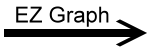﻿ EZ Graph - Tangent Function, Horizontal Translation Variable | Zona Land Education

# EZ Graph - Tangent Function, Horizontal Translation Variable

Click the 'Draw graph' button in the lower left corner of the EZ Graph app. Explanation below.

Here is the conventional notation for this tangent function and the computer language type of notation used with EZ Graph:

 f1(x) = tan(x - h)f1(x) = tan(x - h) f2(x) = tan(x)f2(x) = tan(x)

The two notations are the same for this example.

Custom Search